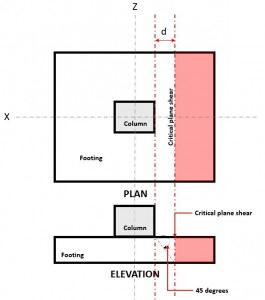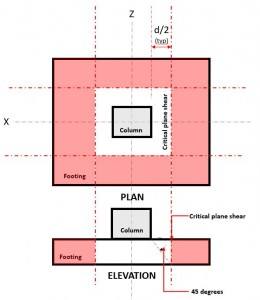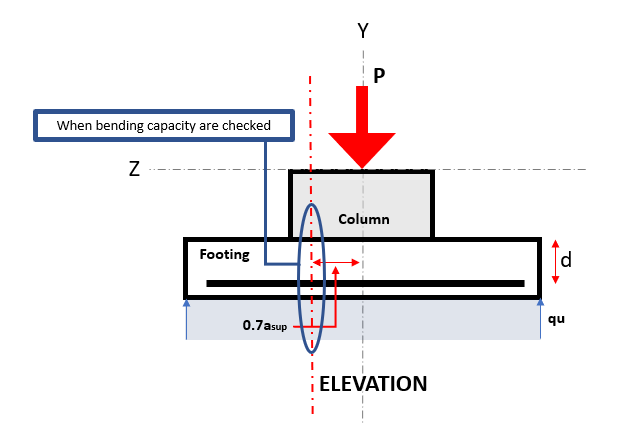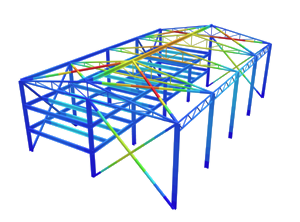SkyCiv Documentation

Your guide to SkyCiv software - tutorials, how-to guides and technical articles

1. Home
2. SkyCiv Foundation
3. Isolated Footings
4. Technical Documentation

## A walk-through of the calculations required to design an isolated footing (AS 3600-09)

The foundation is an essential building system that transfers column and wall forces to the supporting soil. Depending on the soil properties and building loads, the engineer may choose to support the structure on a shallow or deep foundation system³.

SkyCiv Foundation includes the design of isolated footing conforming to the Australian Standards¹.

Want to try SkyCiv’s Foundation Design software? Our tool allows users to perform load-carrying calculations without any download or installation!

## Design of an Isolated Footing

### Dimension Requirements

To determine the dimensions of a isolated footing, service or unfactored loads, such as permanent action (G), imposed action (Q), wind action (Wu), earthquake action (Eu)and Su will be applied using Load Combinations, as defined by AS 3600-09. Whichever Load Combination governs will be considered the design load, and is compared to the allowable soil pressure as shown in Equation 1.

$$\text{q}_{\text{a}} = \frac{\text{P}_{\text{n}}}{\text{A}} \rightarrow$$ Equation 1

where:
qa = allowable soil pressure
Pn = service level design loads
A = foundation area

From Equation 1, qa are interchanged with A.

$$\text{A} = \frac{\text{P}_{\text{n}}}{\text{q}_{\text{a}}} \rightarrow$$ Equation 1a

At this point, the dimensions of the footing can be back-calculated from the required area dimension, A.

### One-way Shear

The one-way shear limit state, also known as flexural shear, is located at a distance “d” from the face of a column, at the Critical Shear Plane (Refer to Figure 1), and is based on AS3600 Clause 8.2.7.1Figure 1. Critical Shear Plane of One-way shear

The One-way Shear Demand  or Vu is calculated assuming the footing is cantilevered away from the column where the area is (red) hatched, indicated in Figure 2.

The One-way Shear Capacity or ϕVuc is defined as Ultimate shear strength and calculated using Equation 2 per AS3600-09 Cl  8.2.7.1.

$$\phi \text{V}_{uc} = \phi \beta_{1} \times \beta_{2} \times \beta_{3} \times b_{v} \times d_{o} \times f_{cv} \times A_{st}^{\frac{2}{3}} \rightarrow$$ Equation 2 (AS3600 Eq. 8.2.7.1

where:
ϕ = shear design factor
β1= 1.1(1.6 – do/1000) ≥ 1.1 or 1.1(1.6(1-do/1000) ≥ 0.8
β2 = 1, for members subject to pure bending; or
=1-(N*/3.5Ag) ≥ 0 for member subject to axial tension; or
=1-(N*/14Ag)   for members subject to axial compression
β3 = 1, or may be taken as –
2do/av but not greater than 2
av = distance for the section at which shear is being considered to the face of the nearest support
fcv = f’c1/3 ≤ 4 MPa
Ast = cross-sectional area of longitudinal reinforcement

Shear Demand and Shear Capacity must meet the following equation to meet the design requirements of AS 3600-09:

$$\text{V}_{\text{u}} \leq \phi\text{V}_{\text{uc}} \rightarrow$$ Equation 3 (per AS3600 Cl. 8.2.5)

SkyCiv Foundation, in compliance of Equation 3, calculates the one-way shear unity ratio (Equation 4) by taking Shear Demand over Shear Capacity.

$$\text{Unity Ratio} = \frac{\text{Shear Demand}}{\text{Shear Capacity}} \rightarrow$$ Equation 4

### Two-way Shear

The Two-way Shear limit state, also known as punching shear, extends it critical section to a distance “d/2” from the face of the column and around the perimeter of the column. The Critical Shear Plane is located at that section of the footing (Refer to Figure 2) based AS3600 Clause 9.2.3(a).Figure 2. Critical Shear Plane of Two-way shear

The Two-way Shear Demand or Vu occurs at the Critical Shear Plane, located a distance of “d/2” where the (red) hatched area, indicated in Figure 2.

The Two-way Shear Capacity or ϕVuo is defined as Ultimate shear strength and calculated using Equation 5 based AS3600 Clause 9.2.3

$$\phi V_{uo} = \phi \times u \times d_{om} \left( f_{cv} + 0.3 \sigma_{cp} \right) \rightarrow$$ Equation 5 (AS3600 Cl. 9.2.3(1)

where:
fcv = 0.17(1 + 2/βh) √f’c ≤ 0.34√f’c
σcp = value of corner, edge and internal columns
dom = mean value of do, averaged around the critical shear perimeter
βh  = ratio of length of column at Z-axis over X-axis
u = length of the critical shear perimeter

Shear Demand and Shear Capacity must meet the following equation to meet the design requirements of AS 3600-09:

$$\text{V}_{\text{u}} \leq \phi\text{V}_{\text{uo}} \rightarrow$$ Equation 6  (per AS3600 Cl. 8.2.5)

SkyCiv Foundation, in compliance of Equation 6, calculates the two-way shear unity ratio (Equation 7) by taking Shear Demand over Shear Capacity.

$$\text{Unity Ratio} = \frac{\text{Shear Demand}}{\text{Shear Capacity}} \rightarrow$$ Equation 7

### Flexure

In a isolated footing, the upward soil pressure causes two-way bending with tensile stresses at the bottom surface. Bending moments are calculated in each direction at sections 0.7asup distance from the centre of the column, where asup is half the width of the column.Figure 3. Critical Flexure Section

The Flexural limit state occurs at the Critical Flexure Section, located 0.7asup from the centre of the footing (Refer to Figure 3).

The Flexural Demand or Mu is located at the Critical Flexure Section indicated in Figure 3, and is calculated using Equation 8.

$$\text{M}^{*}= q_{u} \times D_{f} \times \left( \frac{ \frac{b_{f} – b_{c}}{2} }{2} \right)^{2} \rightarrow$$ Equation 8

The Flexural Capacity or ϕMn is calculated using Equation 9.

$$M_{n} = A_{st} \times f_{sy} \times d \times \left(1- \frac{0.5}{\alpha_{s}} \times \frac{A_{st} \times f_{sy}}{b \times d \times f’_{c}} \right) \rightarrow$$ Equation 9

where:
ϕ = flexural design factor
b = footing dimension parallel x-axis, in or mm
d = distance from extreme compression fiber to centroid of longitudinal tension reinforcement, in or mm
Ast = reinforcement area, in2 or mm2
a = depth of equivalent rectangular stress block, in or mm
fsy = reinforcement strength, ksi or MPa

Moment Demand and Moment Capacity must meet the following equation to meet the design requirements of AS 3600-09:

$$\text{M}_{\text{u}} \leq \phi\text{M}_{\text{n}} \rightarrow$$ Equation 10 (per AS3600 Cl. 8.2.5)

SkyCiv Foundation, in compliance of Equation 10, calculates the flexural unity ratio (Equation 11) by taking Flexural Demand over Flexural Capacity.

$$\text{Unity Ratio} = \frac{\text{Flexure Demand}}{\text{Flexure Capacity}} \rightarrow$$ Equation 11

### Reinforcement

The amount of reinforcement required is determined by flexural strength requirements, with minimum reinforcement specified in Cl. 16.3.1.

$$\rho_{ \text{min} } = 0.19 \times \frac{D}{d}^{2} \times \frac{f’_{ct.f} }{ f_{sy} } \rightarrow$$ Equation 12

The area of steel can be determined with the following equation:

$$\rho = \frac{ 2.7 \times M^{*} }{ d^{2} } \text{ or } \text{A}_{\text{st}} = \frac{ \text{M}^{*} }{ 370 \times \text{d} } \rightarrow$$ Equation 13

As advised by AS 3600-09, a minimum concrete cover of 60 mm for footing is recommended.Albert Pamonag
Structural Engineer, Product Development
B.S. Civil Engineering

## References

1. Council of Standards Australia. (2009) Australian Standard AS3600-2009.
2. SJ Foster, AE Kilpatrick & RF Warner. (2011) Reinforced Concrete Basics 2nd Edition.
3. Taylor, Andrew, et al. The Reinforced Concrete Design Handbook: a Companion to ACI-318-14. American Concrete Institute, 2015.
4. YC Loo & SH Chowdhury. (2013) Reinforced & Prestressed Concrete.

Tags , ,